# User:IssaRice/Proof that assumes the trick

Many proofs in mathematics depend on one or two "tricks". Some proofs (or ways of writing the proof) seem to deliberately hide or assume the trick so that the proof, while valid, is not very useful. (To the expert mathematician, the proof is obvious/they could have done it themselves, so what is the point of writing the proof? To the novice, the trick is assumed even if one might not know it; but the trick is what makes the proof work, so what is the point of seeing a proof that does not explain it?)

Consider Pugh's proof of the product rule for differentiation:

Since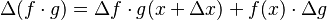$\Delta(f\cdot g) = \Delta f\cdot g(x + \Delta x) + f(x) \cdot \Delta g$, continuity of$g$ at$x$ implies that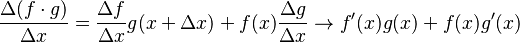$\frac{\Delta(f \cdot g)}{\Delta x} = \frac{\Delta f}{\Delta x} g(x+\Delta x) + f(x)\frac{\Delta g}{\Delta x} \to f'(x)g(x) + f(x)g'(x)$

What Pugh means is this:

On the one hand,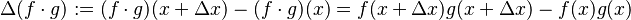$\Delta(f\cdot g) := (f\cdot g)(x + \Delta x) - (f\cdot g)(x) = f(x + \Delta x)g(x + \Delta x) - f(x)g(x)$.

We also have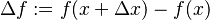$\Delta f := f(x + \Delta x) - f(x)$ and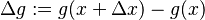$\Delta g := g(x+\Delta x) - g(x)$ so on the other hand we have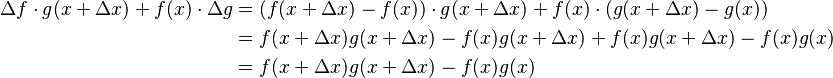\begin{align}\Delta f\cdot g(x + \Delta x) + f(x) \cdot \Delta g &= (f(x + \Delta x) - f(x))\cdot g(x + \Delta x) + f(x) \cdot (g(x+\Delta x) - g(x)) \\ &= f(x + \Delta x)g(x + \Delta x) - f(x)g(x + \Delta x) + f(x)g(x+\Delta x) - f(x)g(x) \\ &= f(x + \Delta x)g(x + \Delta x) - f(x)g(x)\end{align}

so the two are indeed equal.

What is the "trick" in this proof? Well, how would we have discovered that$\Delta(f\cdot g) = \Delta f\cdot g(x + \Delta x) + f(x) \cdot \Delta g$ if we didn't have Pugh to tell us? The trick is to add and subtract the same thing. We have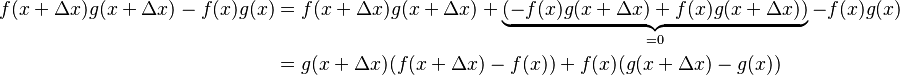\begin{align}f(x + \Delta x)g(x + \Delta x) - f(x)g(x) &= f(x + \Delta x)g(x + \Delta x) + \underbrace{(-f(x)g(x + \Delta x) +f(x)g(x + \Delta x))}_{=0} - f(x)g(x) \\ &= g(x+\Delta x)(f(x+\Delta x) - f(x)) + f(x)(g(x+\Delta x) - g(x))\end{align}

And this is exactly the equality that Pugh gives.

I think what Pugh intended is for the reader to write out the expression on the right and try to simplify it; then they would see the terms canceling out and would see the trick that way. You might even complain that my "discovery" of the trick is just writing out this process in the reverse order. That is certainly literally true, but I think explicitly calling attention to the trick and talking about it adds it to the student's "tricks repository" so that they are more likely to be able to use the trick in their own proofs. In the opposite direction, it's just a routine cancellation and you would just forget about it after the proof.

Here is another example, although I'm actually not sure if this is the same phenomenon (it might be something a little different).

From Axler's Measure, Integration & Real Analysis, theorem 1.5, which shows in part that a finer partition increases the lower Riemann sum.

To prove the first inequality [$L(f,P,[a,b]) \leq L(f,P',[a,b])$], suppose$P$ is the partition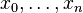$x_0, \ldots, x_n$ and$P'$ is the partition$x_0', \ldots, x_N'$ of$[a,b]$. For each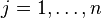$j = 1, \ldots, n$, there exist$k \in \{0, \ldots, N-1\}$ and a positive integer$m$ such that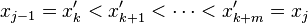$x_{j-1} = x_k' < x_{k+1}' < \cdots < x_{k+m}' = x_j$. We have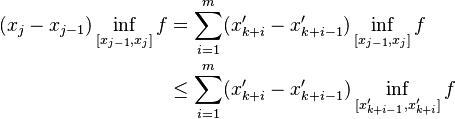\begin{align}(x_j - x_{j-1}) \inf_{[x_{j-1},x_j]} f &= \sum_{i=1}^m (x_{k+i}' - x_{k+i-1}') \inf_{[x_{j-1},x_j]} f \\ &\leq \sum_{i=1}^m (x_{k+i}' - x_{k+i-1}') \inf_{[x_{k+i-1}',x_{k+i}']} f\end{align} The inequality above implies that$L(f,P,[a,b]) \leq L(f,P',[a,b])$.

My first problem with the proof is that it starts off with a bunch of crappy and useless notation. In the first two sentences, Axler has given us four integer variables: n, N, k, and m. Like, wtf??? and it's not even actually helpful for understanding the proof. this just hurts the reader's working memory for no good reason. And the reason he wants to use all these variables is for the sake of perceived generality. He wants to make sure you know that, yes, P and P' have fully general lengths, and that, yes, between each two points in the coarser partition, there can be an arbitrary (finite!) number of inserted points that make the partition finer. i get the motivation for doing this, but it really does not help for getting the point of the proof across.

My second problem with the proof is that the main point of the proof is obscured in a display-style equation. Where is the main point of the proof? Well, it's the part in the display-style equation that does the$\leq$. The ONLY important part of this proof is where it changes from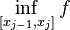$\inf_{[x_{j-1},x_j]} f$ to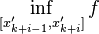$\inf_{[x_{k+i-1}',x_{k+i}']} f$. but what does this change mean? axler sure isn't going to tell you... The important point is this: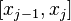$[x_{j-1},x_j]$ is a superset of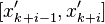$[x_{k+i-1}',x_{k+i}']$ for each$i$.

i want to be clear that my complaint is NOT "this proof is too terse :(". what i am complaining about is that axler is happy to pay the price of setting up a bunch of useless notation, and yet when it comes to actually getting to the point of the proof, he obscures it. if he had just said "this proof is real obvious y'all" i would have been fine with it. (he does this for theorem 1.8 for example.)